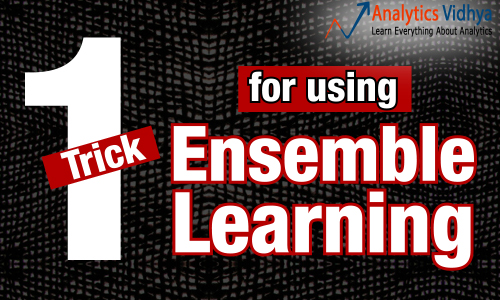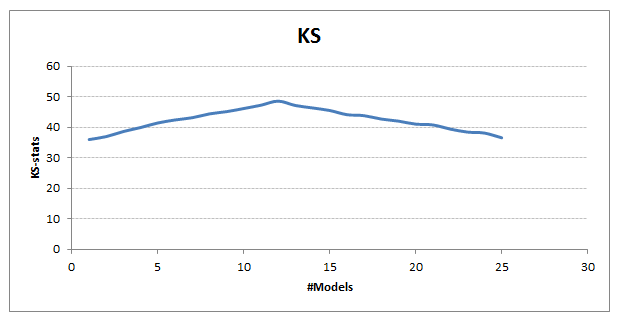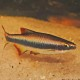Home » Powerful ‘Trick’ to choose right models in Ensemble Learning

# Powerful ‘Trick’ to choose right models in Ensemble Learning

## Introduction

I hope you’ve followed my previous articles on ensemble modeling. In this article, I’ll share a crucial trick helpful to build models using ensemble learning. This trick will teach you,

‘How to choose the right models for your ensemble process?’

Imagine the following scenario (great, if you can relate to it).

You are working on a classification problem and have built 1000 machine learning models. Each of the model gives you an AUC in the range of 0.7 to 0.75 . Your task is to combine all the models together to build a more stable and more predictive model by taking up simple bag of these models.

To answer this question, let’s try to dig into what are we really looking for in these models.## Our Objective

“We are trying to combine a set of high-performing diverse models to get a model that has higher stability and higher performance.”

In the sentence above, two things are essential to note:

1. Models should be High Performing
2. Models should be Diverse

Now, that we know our model objective, let’s try to quantify both these attributes.

1. Quantitative method to gauge model performance: We have various performance metrics which can be tracked in any model. For example: KS stat, AUC-ROC, Lift etc. The selection of performance metric depends on the business problem. Whatever, is your performance metric of interest, the same metric should be used to track your ensemble process.
2. Quantitative method to gauge model diversity: Diversity is an interesting clause and there is no perfect solution to this problem. But, we can introduce diversity through various methods. Most commonly used are Correlation coefficient – Pearson or Spearman, %overlap in the predicted class, bin wise chi-squared value etc.

In this article I’ll will choose KS-stat as the Performance Metric and Pearson Coefficient as the Diversity Metric.## The Algorithm

There is no perfect algorithm to select the right set of variables. However, in this article I have laid down a methodology which I have found extremely effective in finding the right model set. Following is the step by step methodology with the relevant codes :

### Step 1 :  Find the KS of individual models

We start with finding the performance of individual models. You can use following code :

`#I assume train is a table with 1002 columns : column 1 is ID of the row 2:1001 are predictions from individual models and 1002 is the target categorical variable.`
```train_ks <- 1:1000
for (i in 2:1001){
train_ks[i-1] <- max(ks_compute(train[,i],train[,1002]))} #ks_compute is a function whichI have written locally, you can replace it with AUC ROC which is internally built in R.

```

### Step 2: Index all the models for easy access

Let’s try to be effective while referencing our models. Here is a powerful way to do the same:

`sno <- 2:1001`
```train_ks_table <- cbind(sno,train_ks)
train_ks_table <- train_ks_table[order(-train_ks_table[,2]),]
train_order <-c(1,train_ks_table[,1],1002)```
`train_sorted <- train[,train_order]`

### Step 3: Choose the first two models as the initial selection and set  a correlation limit

```models_selected <- colnames(train_sorted)[2:3]
limit_corr <- 0.75```

### Step 4: Iteratively choose all the models which are not highly correlated with any of the any chosen model

Here is where you make the final comparison using the performance and the diversity factor (Pearson Coefficient)

```for (i in 3:1000) {
choose = 1
for (j in 1:length(models_selected)) {
correlation <- cor(train_sorted[,i],train_sorted[,models_selected[j]])
choose <- ifelse(correlation > limit_corr,0,1*choose)
}
if(choose == 1) {
models_selected <- c(models_selected,colnames(train_sorted)[i])
}
}```

Now you have a list of models selected in the vector models_selected.

### Step 5: Time to check the performance of individual sequential combination

Having chosen a sequence of models, now is the time to add each combination and check their performance.

`train_ks_choose <- rep(1,length(models_selected))`
`predictions_train <- apply(train_sorted[,2:3],1,mean)`
```model_considered = 0
for (j in 1:length(models_selected)){
predictions_train <- (model_considered*predictions_train + train_sorted[,models_selected[j]])/(model_considered + 1)
train_ks_choose[j] <- max(ks_compute(predictions_train,train[,462])) #38.49%
model_considered = model_considered + 1
}```
```ks_table <- cbind(train_ks_choose,itv1_ks_choose,itv2_ks_choose)
write.csv(ks_table,"ks_table.csv")

```

### Step 6: Choose the combination where the performance peaks

Here is the plot I get after executing the codeIt is quite clear from the above graph that we see significant benefits by combining around 12 diverse high-performing models. Our final KS goes up to 49 from 36 (individual model maximum).

How about applying this trick to solve real life problem? Register here for our largest ever Hackathon – The D Hack and compete against more than 500 data scientists across the globe and get a chance to win \$300.

## End Notes

Ensemble model gives significant lift over individual models because it is able to combine the positive attributes of multiple models. There is no specific way to combine models together, however I have seen with experience best selections get more intuitive.

Have you tried ensemble learning? If yes, did you see a significant benefit. What method did you use? Share with us your comments/queries/inputs on this article.

### If you like what you just read & want to continue your analytics learning, subscribe to our emails, follow us on twitter or like our facebook page.

You can also read this article on our Mobile APP•Sannmeet Walia says:

Nice Explanation!

•Frederick says:

I have been following all your post and you have been very helpful, especially for beginners like myself. However, it would be very helpful if you could provide the data used. This will enable the learning as we follow each step.

•Lars says:

Interesting article. How would you go from this result to predicting a new set of data?

•Tavish Srivastava says:

You make individual model predictions and then apply the weights found from this simulation.

•Doug Dame says:

Tavish, I’m belatedly returning to this excellent article/posting with a question:

Have you tested to see how your “find the diverse models” approach compares to an alternative of simply using an elastic net (or LARS) regression on the train table you have (that contains all 1000 predictions as columns, to essentially regularize the non-useful models right out of the ensemble solution?

I’m thinking that we’d get just about the same result, but a bit simpler and faster.

As the tabloids by the cash register in the grocery store say, “Inquiring Minds Want To Know.” 🙂

HTH

•avaneesh kumar says:

can you elaborate on the concept?

•avaneesh kumar says:

So informative, thank you Tavish for such an epiphany.
Doug Dame, can you elaborate? I want to know how you thought about the same problem.